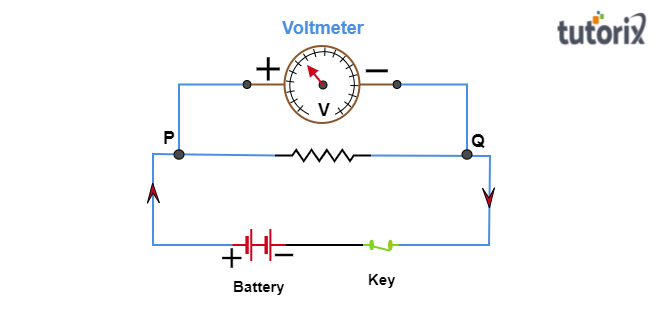# How is a voltmeter connected in the circuit to measure the potential difference between two points?

Voltmeter:
A voltmeter is a device that is used to find the potential difference between any two points in an electric circuit.

A voltmeter can't be connected in series:
A voltmeter can not be connected in series as it has a very high resistance that will increase the total resistance of the whole circuit and this highly increased resistance of the circuit will not let the current pass through the circuit.

A voltmeter is connected in parallel:
A voltmeter is a connected parallel to the two points across which we are finding the potential difference.

Diagram
In the following diagram, a voltmeter is a connected parallel to $PQ$ to measure the potential difference between two points $P$ and $Q$. When the circuit is closed by using key$(switch)$, the current starts flowing through the circuit from the positive to the negative terminal. the needle of the voltmeter shows the potential difference between the points $P$ and $Q$.